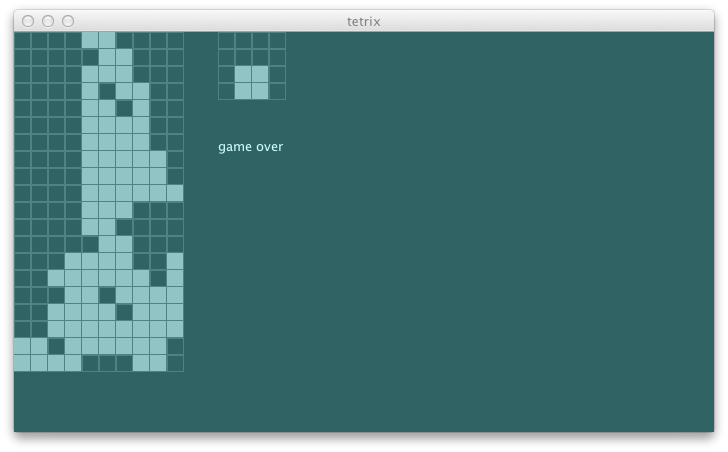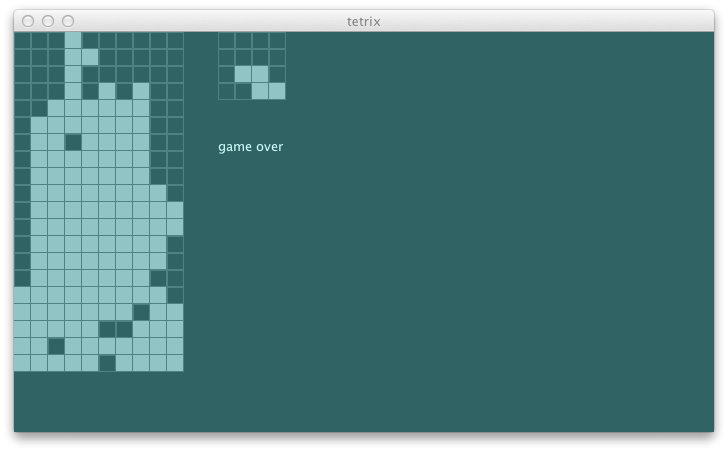# tetrix in Scala: day 8

Yesterday we hooked up our tetrix-solving agent to an actor to take control of the game. Thus far the way it handled the game looked neither rational nor intelligent. After seeing many of the moves evaluated to 0.0 score including the heuristic penalties, I had two sneaking suspicions.

First, `Drop` was never a good choice. Especially given the shallow search tree, selecting `Drop` seemed premature. Since the gravitational tick is going to take care of the downard movement anyway, I decided to ignore it when the agent thinks the best move is `Drop`:

```  def receive = {
case BestMove(s: GameState) =>
val message = agent.bestMove(s)
if (message != Drop) stageActor ! message
}```

### buggy penalty

Second suspicion was that there was something wrong with the penalty calculation. Let's add more test:

```    """penalize having gaps between the columns"""          ! utility4^
...
def utility4 = {
val s = newState(Seq(
(0, 0), (0, 1), (0, 2), (0, 3), (0, 4), (0, 5), (0, 6))
map { Block(_, TKind) }, (10, 20), TKind :: TKind :: Nil)
agent.utility(s) must_== -36.0
} and {
val s = newState(Seq((1, 0), (1, 1), (2, 1), (2, 2))
map { Block(_, ZKind) }, (10, 20), TKind :: TKind :: Nil)
agent.utility(s) must_== -13.0
}```

And it fails.

```[error] x penalize having gaps between the columns
[error]    '-4.0' is not equal to '-13.0' (AgentSpec.scala:35)```

Before we go into the REPL, we can save some typing by adding the following to `build.scala`:

```initialCommands in console := """import com.eed3si9n.tetrix._
|import Stage._""".stripMargin```

After reloading, type in `console` from sbt shell and open the REPL:

```[info]
import com.eed3si9n.tetrix._
import Stage._
Welcome to Scala version 2.9.2 (Java HotSpot(TM) 64-Bit Server VM, Java 1.6.0_33).
Type in expressions to have them evaluated.

scala> move // hit tab key
moveLeft    moveRight ```

Yes, the tab key completion works in the REPL.

```scala>     val s = newState(Seq((1, 0), (1, 1), (2, 1), (2, 2))
map { Block(_, ZKind) }, (10, 20), TKind :: TKind :: Nil)
s: com.eed3si9n.tetrix.GameState = GameState(List(Block((1,0),ZKind), Block((1,1),ZKind), Block((2,1),ZKind), Block((2,2),ZKind), Block((4,18),TKind), Block((5,18),TKind), Block((6,18),TKind), Block((5,19),TKind)),(10,20),Piece((5.0,18.0),TKind,List((-1.0,0.0), (0.0,0.0), (1.0,0.0), (0.0,1.0))),Piece((2.0,1.0),TKind,List((-1.0,0.0), (0.0,0.0), (1.0,0.0), (0.0,1.0))),List(),ActiveStatus,0)

scala>     val heights = s.unload(s.currentPiece).blocks map {
_.pos} groupBy {_._1} map { case (k, v) => (k, v.map({_._2}).max) }
heights: scala.collection.immutable.Map[Int,Int] = Map(1 -> 1, 2 -> 2)

scala>     val gaps = (0 to s.gridSize._1 - 2).toSeq map { x =>
heights.getOrElse(x, 0) - heights.getOrElse(x + 1, 0) } filter {_ > 1}
gaps: scala.collection.immutable.IndexedSeq[Int] = Vector(2)```

Off by one error! Did you catch this? The lowest coordinate is `0`, yet I am returning `0` as the default.

Also I am throwing out the negative numbers. The correct `gap` should be:

```scala>     val gaps = (0 to s.gridSize._1 - 2).toSeq map { x =>
heights.getOrElse(x, -1) - heights.getOrElse(x + 1, -1) } filter {math.abs(_) > 1}
gaps: scala.collection.immutable.IndexedSeq[Int] = Vector(-2, 3)```

I didn't catch this because my hand calculated value of -36.0 was also incorrect, which should have been -49.0. Now all tests pass:

`[info] + penalize having gaps between the columns`

It feels more logical now, but the penalty isn't nudging the game to actual scoring or creating the right set up. First, I want to separate the reward component and penalty component of the utility function so it's easier to test. Second, instead of the gaps, let's try penalizing the heights in general.

```  "Penalty function should"                                 ^
"""penalize having blocks stacked up high"""            ! penalty1^
...
def penalty1 = {
val s = newState(Seq(
(0, 0), (0, 1), (0, 2), (0, 3), (0, 4), (0, 5), (0, 6))
map { Block(_, TKind) }, (10, 20), TKind :: TKind :: Nil)
agent.penalty(s) must_== 49.0
} and {
val s = newState(Seq((1, 0))
map { Block(_, ZKind) }, (10, 20), TKind :: TKind :: Nil)
agent.penalty(s) must_== 1.0
}```

Here's the new `penalty`:

```  def penalty(s: GameState): Double = {
val heights = s.unload(s.currentPiece).blocks map {
_.pos} groupBy {_._1} map { case (k, v) => v.map({_._2 + 1}).max }
heights map { x => x * x } sum
}```

Another thing I want to tweak is the balance between the reward and penalty. Currently the incentive of deleting the line is too little compared to avoiding the penalty.

```  def utility(state: GameState): Double =
if (state.status == GameOver) minUtility
else reward(state) - penalty(state) / 10.0```

This at least made the agent delete a line in the following game:### pruning the search tree

Pruning allows us to ignore portion of the search tree that make no difference to the final choice.

This concept applies here because without control the search tree grows exponentially.

1. We can reduce the branching factor from five to tree by omitting `Drop` and `Tick`. We already pretend that the current pieces gets dropped. All we have to do is to evaluate `s0` with `Drop`.
2. Next, we can eliminate `RotateCW` by pre-branching for all four orientations of the current piece. In most cases `RotateCW :: MoveLeft :: RotateCW :: Drop :: Nil` and `RotateCW :: RotateCW :: MoveLeft :: Drop :: Nil` brings us to the same state.
3. We can get rid of `MoveLeft` by moving the current piece as left as it can upfront.

Potentially a piece can have four orientations and nine x-position. Thus, exponential tree search tree can now be approximated by a constant size 36.

First, we can list out the possible orientations based on the `PieceKind`:

```  private[this] def orientation(kind: PieceKind): Int = {
case IKind => 2
case JKind => 4
case LKind => 4
case OKind => 1
case SKind => 2
case TKind => 4
case ZKind => 2
}```

Next, given a state calculate how many times can the agent hit left or right using the REPL.

```scala> val s = newState(Nil, (10, 20), TKind :: TKind :: Nil)
s: com.eed3si9n.tetrix.GameState = GameState(List(Block((4,18),TKind), Block((5,18),TKind), Block((6,18),TKind), Block((5,19),TKind)),(10,20),Piece((5.0,18.0),TKind,List((-1.0,0.0), (0.0,0.0), (1.0,0.0), (0.0,1.0))),Piece((2.0,1.0),TKind,List((-1.0,0.0), (0.0,0.0), (1.0,0.0), (0.0,1.0))),List(),ActiveStatus,0)

scala> import scala.annotation.tailrec
import scala.annotation.tailrec

scala> @tailrec def leftLimit(n: Int, s: GameState): Int = {
val next = moveLeft(s)
if (next.currentPiece.pos == s.currentPiece.pos) n
else leftLimit(n + 1, next)
}
leftLimit: (n: Int, s: com.eed3si9n.tetrix.GameState)Int

scala> leftLimit(0, s)
res1: Int = 4```

Make the same one for right, and we have `sideLimit` method:

```  private[this] def sideLimit(s0: GameState): (Int, Int) = {
@tailrec def leftLimit(n: Int, s: GameState): Int = {
val next = moveLeft(s)
if (next.currentPiece.pos == s.currentPiece.pos) n
else leftLimit(n + 1, next)
}
@tailrec def rightLimit(n: Int, s: GameState): Int = {
val next = moveRight(s)
if (next.currentPiece.pos == s.currentPiece.pos) n
else rightLimit(n + 1, next)
}
(leftLimit(0, s0), rightLimit(0, s0))
}```

These should be enough to build `actionSeqs`:

```  "ActionSeqs function should"                              ^
"""list out potential action sequences"""               ! actionSeqs1^
...
def actionSeqs1 = {
val s = newState(Nil, (10, 20), TKind :: TKind :: Nil)
val seqs = agent.actionSeqs(s)
seqs.size must_== 32
}```

Stub it out:

`  def actionSeqs(s0: GameState): Seq[Seq[StageMessage]] = Nil`

The test fails as expected:

```[info] ActionSeqs function should
[error] x list out potential action sequences
[error]    '0' is not equal to '32' (AgentSpec.scala:15)```

Here's the implementation:

```  def actionSeqs(s0: GameState): Seq[Seq[StageMessage]] = {
val rotationSeqs: Seq[Seq[StageMessage]] =
(0 to orientation(s0.currentPiece.kind) - 1).toSeq map { x =>
}
val translationSeqs: Seq[Seq[StageMessage]] =
sideLimit(s0) match {
case (l, r) =>
((1 to l).toSeq map { x =>
}) ++
Seq(Nil) ++
((1 to r).toSeq map { x =>
})
}
for {
r <- rotationSeqs
t <- translationSeqs
} yield r ++ t
}```

We can see the output using REPL:

```scala> val s = newState(Nil, (10, 20), TKind :: TKind :: Nil)
s: com.eed3si9n.tetrix.GameState = GameState(List(Block((4,18),TKind), Block((5,18),TKind), Block((6,18),TKind), Block((5,19),TKind)),(10,20),Piece((5.0,18.0),TKind,List((-1.0,0.0), (0.0,0.0), (1.0,0.0), (0.0,1.0))),Piece((2.0,1.0),TKind,List((-1.0,0.0), (0.0,0.0), (1.0,0.0), (0.0,1.0))),List(),ActiveStatus,0)

scala> val agent = new Agent
agent: com.eed3si9n.tetrix.Agent = com.eed3si9n.tetrix.Agent@649f7367

scala> agent.actionSeqs(s)
res0: Seq[Seq[com.eed3si9n.tetrix.StageMessage]] = Vector(List(MoveLeft), List(MoveLeft, MoveLeft), List(MoveLeft, MoveLeft, MoveLeft), List(MoveLeft, MoveLeft, MoveLeft, MoveLeft), List(), List(MoveRight), List(MoveRight, MoveRight), List(MoveRight, MoveRight, MoveRight), List(RotateCW, MoveLeft), List(RotateCW, MoveLeft, MoveLeft), List(RotateCW, MoveLeft, MoveLeft, MoveLeft), List(RotateCW, MoveLeft, MoveLeft, MoveLeft, MoveLeft), List(RotateCW), List(RotateCW, MoveRight), List(RotateCW, MoveRight, MoveRight), List(RotateCW, MoveRight, MoveRight, MoveRight), List(RotateCW, RotateCW, MoveLeft), List(RotateCW, RotateCW, MoveLeft, MoveLeft), List(RotateCW, RotateCW, MoveLeft, MoveLeft, MoveLeft), List(RotateCW, RotateCW, MoveLeft, MoveLeft, MoveLeft, MoveLeft), List(RotateCW, RotateCW),...```

Note one of the action sequences is `List()`, which evaluates the current state. All tests pass too:

```[info] ActionSeqs function should
[info] + list out potential action sequences```

We can now rewrite `bestMove` using `actionSeqs`:

```  def bestMove(s0: GameState): StageMessage = {
var retval: Seq[StageMessage] = Nil
var current: Double = minUtility
actionSeqs(s0) foreach { seq =>
val ms = seq ++ Seq(Drop)
val u = utility(Function.chain(ms map {toTrans})(s0))
if (u > current) {
current = u
retval = seq
} // if
}
println("selected " + retval + " " + current.toString)
}```

Now let's add more spec. How about having a single gap open at `(0, 8)` such that it requires several rotations and a bunch of `MoveRight`s? This is something our agent would have not solved before.

```  "Solver should"                                           ^
"""pick MoveLeft for s1"""                              ! solver1^
"""pick Drop for s3"""                                  ! solver2^
"""pick RotateCW for s5"""                              ! solver3^
...
def s5 = newState(Seq(
(0, 0), (1, 0), (2, 0), (3, 0), (4, 0), (5, 0), (6, 0),
(7, 0), (9, 0))
map { Block(_, TKind) }, (10, 20), ttt)
def solver3 =
agent.bestMove(s5) must_== RotateCW```

All green. Now let's run the swing UI to see how it looks:```[info] selected List(RotateCW, MoveLeft, MoveLeft, MoveLeft, MoveLeft) 1.4316304877998318
[info] selected List(MoveLeft, MoveLeft, MoveLeft, MoveLeft) 1.4316304877998318
[info] selected List(MoveLeft, MoveLeft, MoveLeft) 1.4316304877998318
[info] selected List(MoveLeft, MoveLeft) 1.4316304877998318
[info] selected List() 1.4108824377664941```

It is rather short-sighted in its moves, but I am starting to see the glimpse of rationality. We'll pick it up from here tomorrow.

```\$ git fetch origin
\$ git co day8 -b try/day8
\$ sbt "project swing" run```